# IMPLICATION OF A MEASUREMENT AT LHC OF THE

• Slides: 16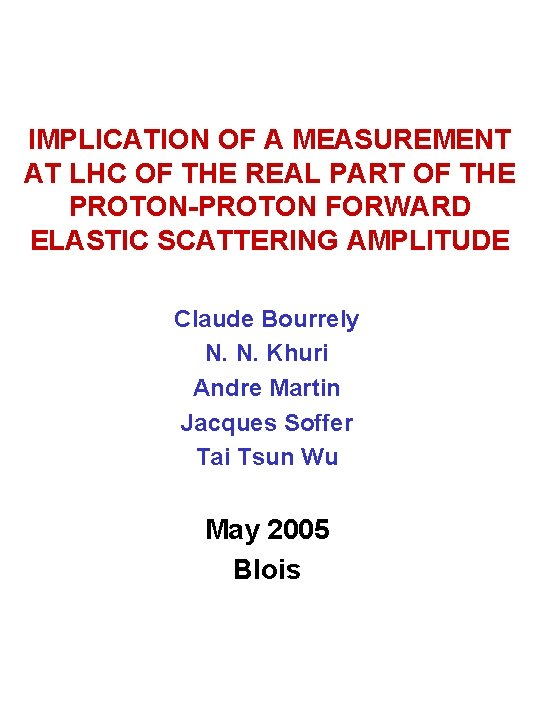IMPLICATION OF A MEASUREMENT AT LHC OF THE REAL PART OF THE PROTON-PROTON FORWARD ELASTIC SCATTERING AMPLITUDE Claude Bourrely N. N. Khuri Andre Martin Jacques Soffer Tai Tsun Wu May 2005 Blois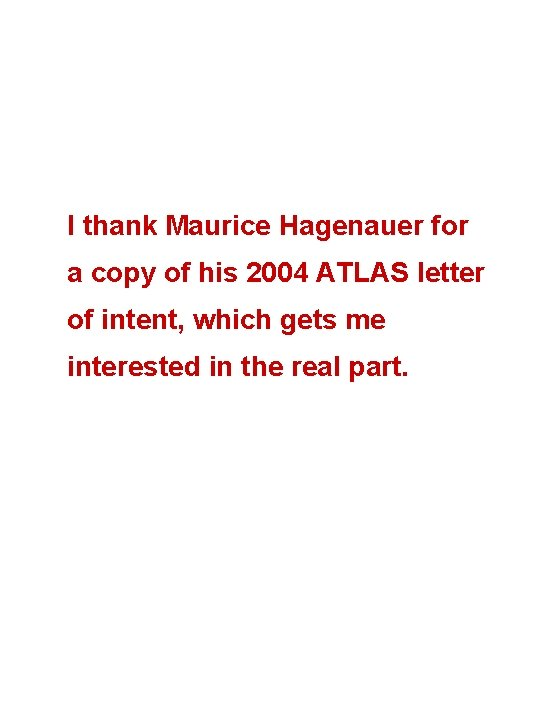I thank Maurice Hagenauer for a copy of his 2004 ATLAS letter of intent, which gets me interested in the real part.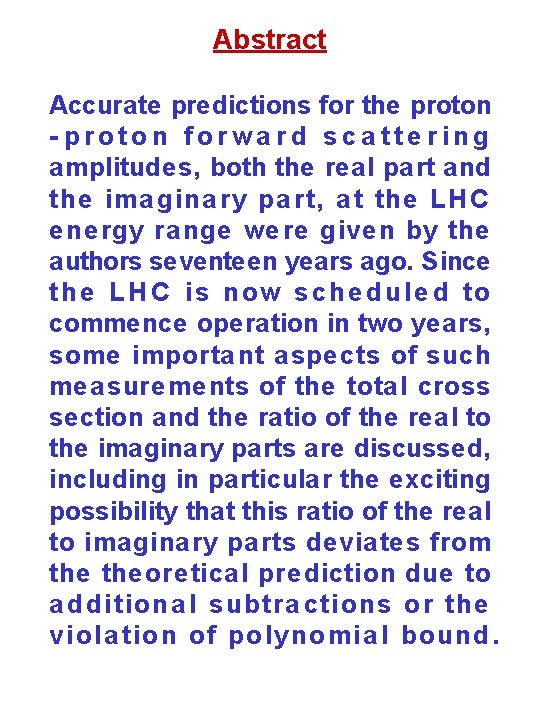Abstract Accurate predictions for the proton - proton forwa rd sca tte ring amplitudes, both the real part and the ima gina ry pa rt, a t the LH C e ne rgy ra nge we re give n by the authors seventeen years ago. Since the LH C is now sche dule d to commence operation in two years, some importa nt a spe cts of such measurements of the total cross section and the ratio of the real to the imaginary parts are discussed, including in particular the exciting possibility that this ratio of the real to imaginary parts deviates from theoretical prediction due to a dditiona l subtra ctions or the viola tion of polynomia l bound.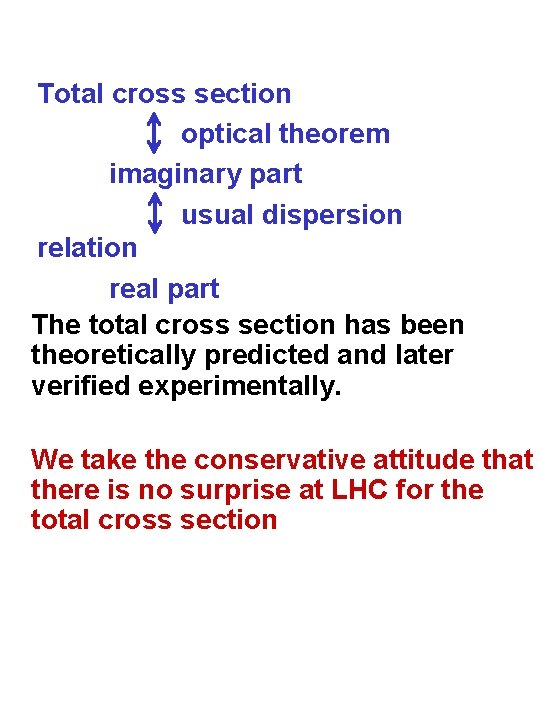Total cross section optical theorem imaginary part usual dispersion relation real part The total cross section has been theoretically predicted and later verified experimentally. We take the conservative attitude that there is no surprise at LHC for the total cross sectionf(s): pp forward scattering amplitude. Apply the usual dispersion relation to Im f(s). Call the result g(s). Then f(s)=g(s)+E(s) Where E(s) is analytic in the entire s plane, and is real when s is real.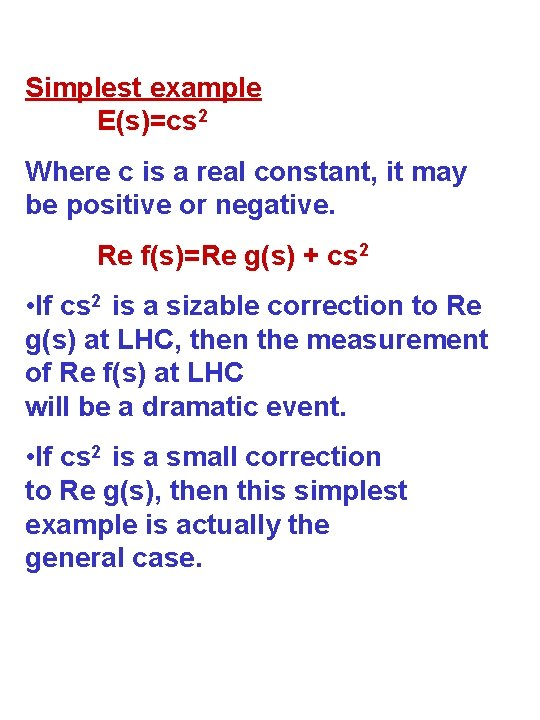Simplest example E(s)=cs 2 Where c is a real constant, it may be positive or negative. Re f(s)=Re g(s) + cs 2 • If cs 2 is a sizable correction to Re g(s) at LHC, then the measurement of Re f(s) at LHC will be a dramatic event. • If cs 2 is a small correction to Re g(s), then this simplest example is actually the general case.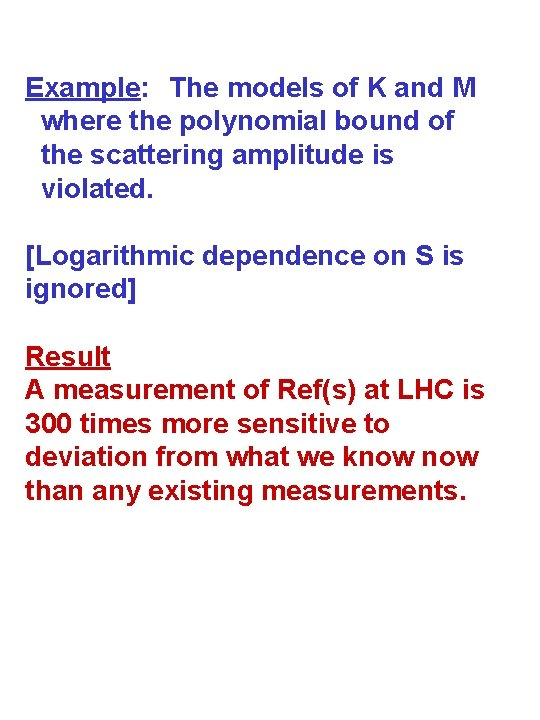Example: The models of K and M where the polynomial bound of the scattering amplitude is violated. [Logarithmic dependence on S is ignored] Result A measurement of Ref(s) at LHC is 300 times more sensitive to deviation from what we know than any existing measurements.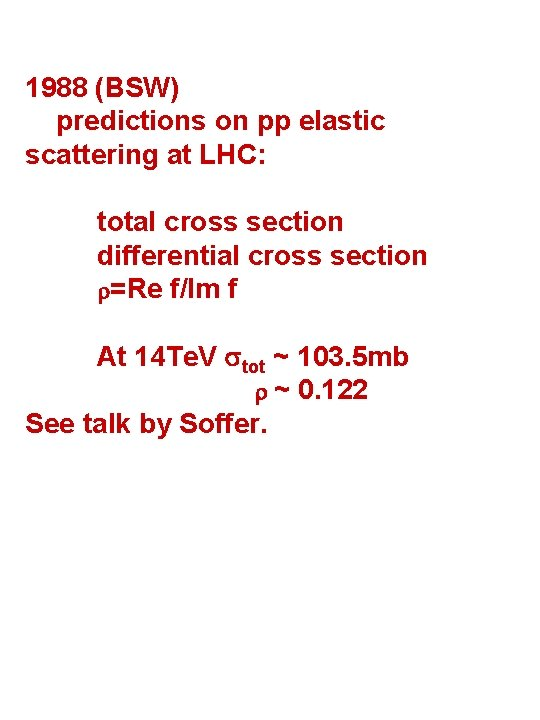1988 (BSW) predictions on pp elastic scattering at LHC: total cross section differential cross section =Re f/Im f At 14 Te. V tot ~ 103. 5 mb ~ 0. 122 See talk by Soffer.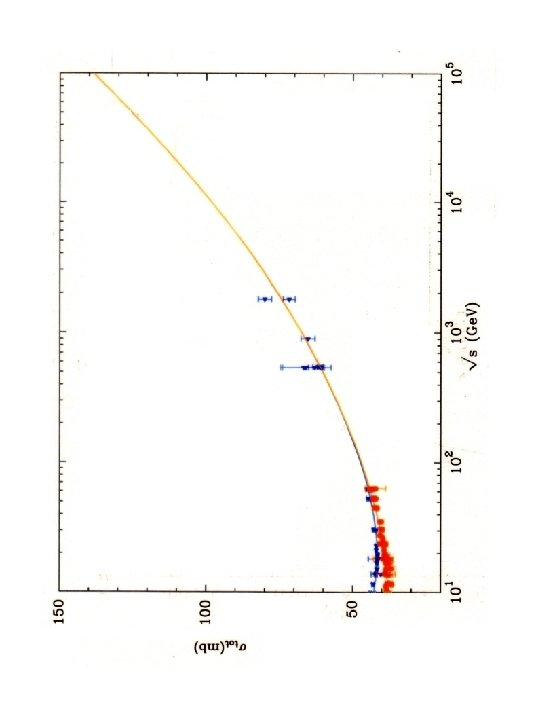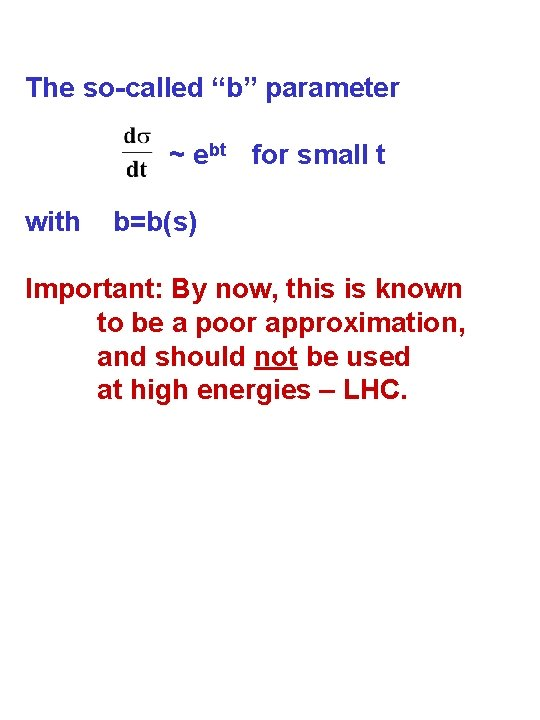The so-called “b” parameter ~ ebt for small t with b=b(s) Important: By now, this is known to be a poor approximation, and should not be used at high energies – LHC.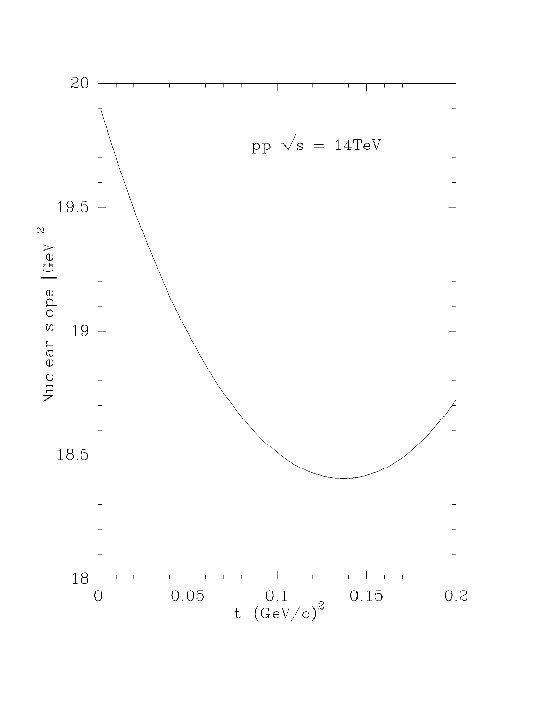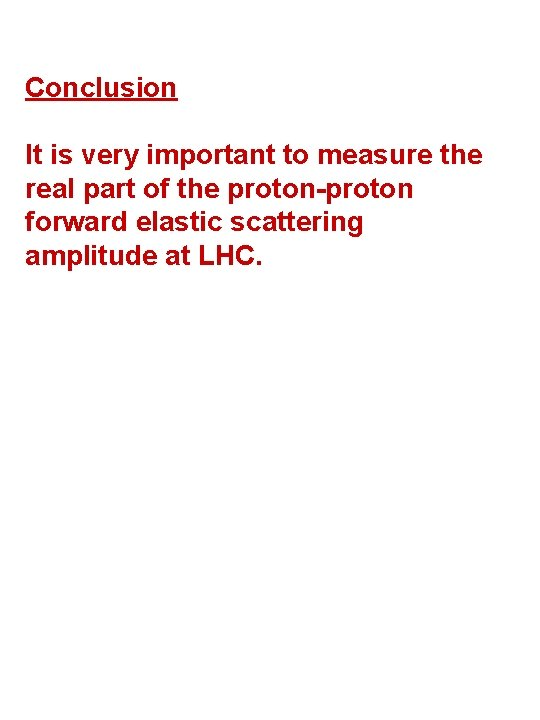Conclusion It is very important to measure the real part of the proton-proton forward elastic scattering amplitude at LHC.